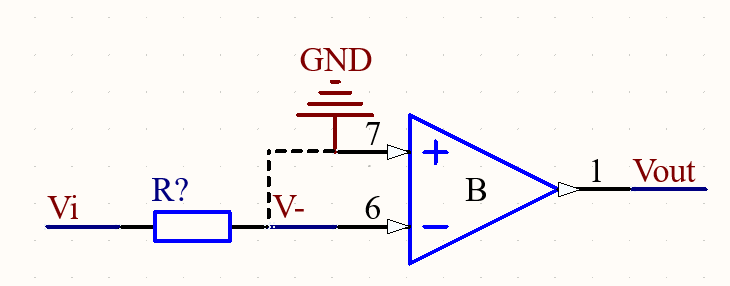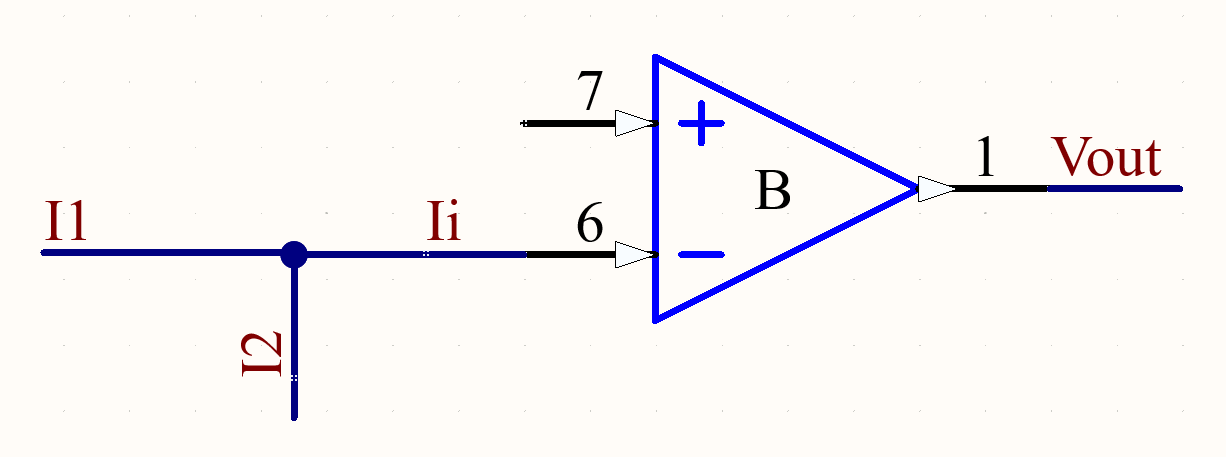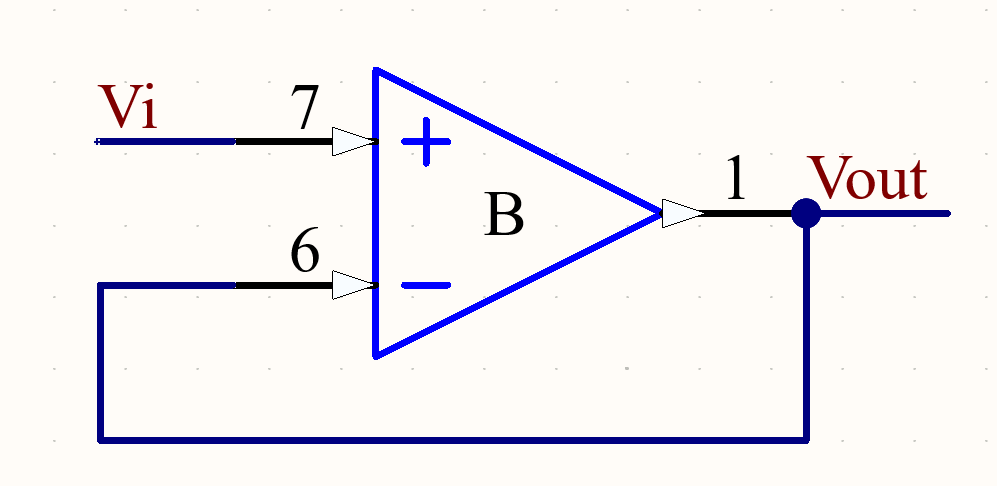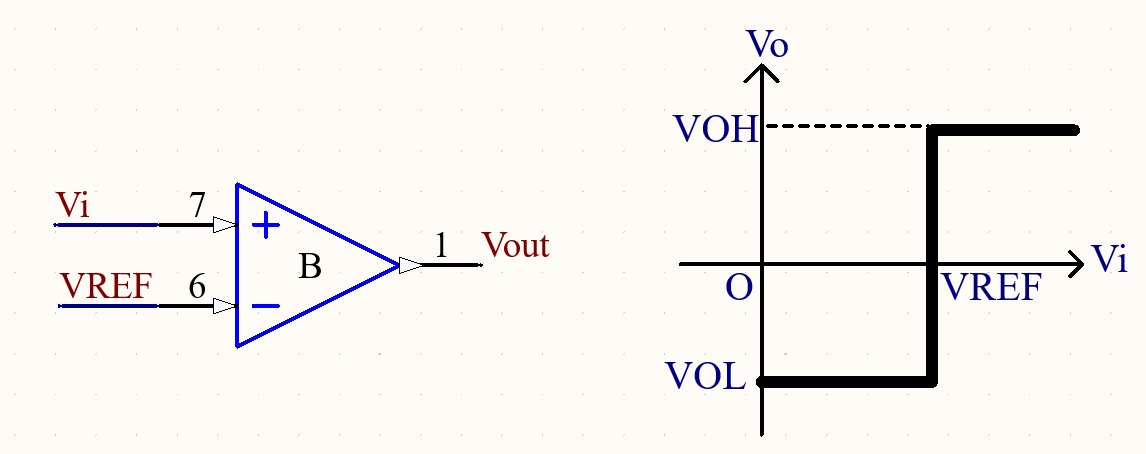# 浅谈集成运放及典型运放电路·信号反向端输入，输出电压Vout与输入电压Vi之间存在反相位关系

·R2是反馈电阻，其作用是引入深度负反馈来稳定电路的工作

·R3是平衡电阻，用来维持集成运放输入级差分电路对称性能，通常R3=R1//R2

由虚短概念：V- V+=0

由虚断概念:  i1 i2

由电位关系:  i1=Vi-V-)/R1 = Vi/R1

i2=V- -Vo)/R2 = -Vo/R2

Vi/R1 = -Vo/R2

Vo = -(R2/R1)Vi·信号同相端输入，输出电压Vout与输入电压Vi之间存在同相位关系

·输入信号通过一个分压电路加到同向端，图中R3R4同相端没有电流

由虚短概念：V- V+

由虚断概念:  i1 i2

由电位关系:  i1=V- -0)/R1 = V+/R1

i2=Vo -V-)/R2 = (Vo-V+)/R2

V+/R1 = (Vo-V+)/R2

Vo = (1+R2/R1)V+

V+=(R4/(R3+R4))Vi

Vo=(1+R2/R1)(R4/(R3+R4))Vi·信号同相端输入

·反相输入端与输出端用导线连接。

由虚短概念可以得到电路输入输出关系：

Vo = V- V+ = Vi

Vo = Vi